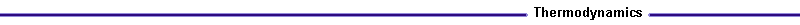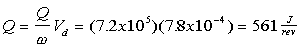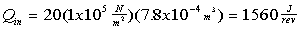What is Qin?Here is one method for determine Qin from given information for an engine. For an automotive engine, the power per liter is given as 3.37x104 W/liter, which is equivalent to 34 MW/m3 after a unit conversion. Doubling this value to include heat transfer results in approximately 60 MW/m3. Next we need to determine the heat input in Joules for one cycle. Given that the engine speed, w, is 5000 rpm: If we are given the bore and the stroke to be: bore = 90 mm = 0.09 m ~ 0.1 m stroke = 80 mm = 0.08m ~ 0.1 m The displacement volume, Vd, can be found from:Which leads to :If we use, Qin = 20P1Vd, at 1atm, we get:This result is much higher than the first method. If we use this last method, the energy density is taken into account. This last method will be used to determine the overall heat input.